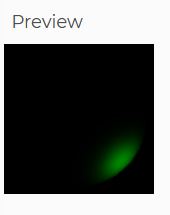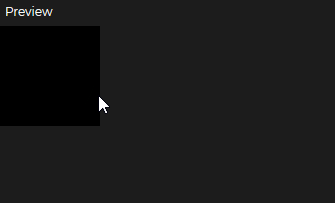Related Articles

# p5.js spotLight() Method

• Last Updated : 04 Mar, 2021

The spotLight() function in p5.js is used to create a spotlight with a given color, position, direction of light, angle and concentration. A scene can have a maximum of 5 spotlights active at one time. The coordinates of the spotlight can be set according to the below-given diagram.

Syntax: This method can be used with multiple parameters depending on the syntax.

```spotLight(v1, v2, v3, x, y, z, rx, ry, rz, [angle], [conc])
spotLight(color, position, direction, [angle], [conc])
spotLight(v1, v2, v3, position, direction, [angle], [conc])
spotLight(color, x, y, z, direction, [angle], [conc])
spotLight(color, position, rx, ry, rz, [angle], [conc])
spotLight(v1, v2, v3, x, y, z, direction, [angle], [conc])
spotLight(v1, v2, v3, position, rx, ry, rz, [angle], [conc])
spotLight(color, x, y, z, rx, ry, rz, [angle], [conc])

```

Parameters: This function accept fifteen parameters as mentioned above and described below:

• v1: It is a number which determines the red or hue value relative to the current color mode.
• v2: It is a number which determines the green or saturation value relative to the current color mode.
• v3: It is a number which determines the blue or brightness value relative to the current mode.
• x: It is the position of the light on the x-axis.
• y: It is the position of the light on the y-axis.
• z: It is the position of the light on the z-axis.
• rx: It is the direction of light in the x-axis.
• ry: It is the direction of light in the y-axis.
• rz: It is the direction of light in the z-axis.
• angle: It provides the angle for light. The default value is PI/3.
• conc: It specifies the concentration value for the light. The default value is 100.
• color: It specifies the color of the light. It can either be color Array, CSS color string, or p5.Color value.
• position: It is a p5.Vector which specifies the position of the light.
• direction: It is a p5.Vector which specifies the direction of the light.

Below examples illustrates the spotLight() function in p5.js:

Example 1: This example show the spotlight at one specific position.

## Javascript

 `function` `setup() {``   ` `  ``// Creating canvas with width``  ``// and height of 100``  ``createCanvas(100, 100, WEBGL);``}`` ` `function` `draw() {`` ` `  ``// Setting backgroud colour``  ``// to black``  ``background(0);``   ` `  ``// Setting the spotlight``  ``spotLight(0, 250, 0, 20, 20,``            ``100, 0, 0, -1, Math.PI );``  ``noStroke();``   ` `  ``// Drawing a sphere to show``  ``// the spotlight``  ``sphere(40);``}`

Output:Example 2: This example illustrates how to change the spotlight position based on the mouse movement.

## Javascript

 `function` `setup() {``   ` `  ``// Creating canvas with width``  ``// and height to 100``  ``createCanvas(100, 100, WEBGL);``}`` ` `function` `draw() {``  ``// Setting backgroud colour``  ``// to black``  ``background(0);``   ` `  ``// Getting position based``  ``// on mouse movement``  ``let locX = mouseX - width / 2;``  ``let locY = mouseY - height / 2;``   ` `  ``// Setting the spotlight``  ``spotLight(0, 250, 0, locX, locY,``            ``100, 0, 0, -1, Math.PI );``  ``noStroke();``   ` `  ``// Drawing a sphere to show ``  ``// the spotlight``  ``sphere(40);``}`

Output:My Personal Notes arrow_drop_up